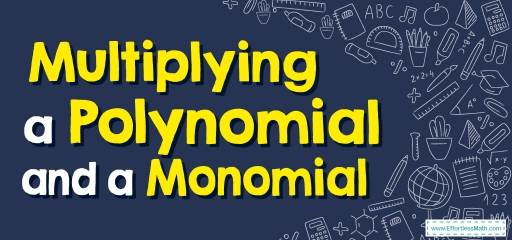# How to Multiply a Polynomial and a Monomial? (+FREE Worksheet!)

Learn how to multiply monomials to polynomials using the distributive property and the multiplication property of exponents.## Step by step guide to Multiplying a Polynomial and a Monomial

• When multiplying monomials, use the product rule for exponents.
• When multiplying a monomial by a polynomial, use the distributive property.
$$\color{blue}{a×(b+c)=a×b+a×c}$$

Best Math for Grade 8 Resource

### Multiplying a Polynomial and a Monomial – Example 1:

Multiply expressions. $$2x(-2x+4)=$$

Solution:

Use Distributive Property: $$\color{blue}{a \ (b \ + \ c)=ab \ + \ ac}$$

Then: $$2x(-2x+4)=-4x^2+8x$$

### Multiplying a Polynomial and a Monomial – Example 2:

Multiply expressions. $$-2x(3x^2+4y^2 )=$$

Solution:

Use Distributive Property: $$\color{blue}{a \ (b \ + \ c)=ab \ + \ ac}$$

Then: $$-2x(3x^2+4y^2 )=-6x^3-8xy^2$$

### Multiplying a Polynomial and a Monomial – Example 3:

Multiply expressions. $$-4x(5x+9)=$$

Solution:

Use Distributive Property: $$\color{blue}{a \ (b \ + \ c)=ab \ + \ ac}$$

Then: $$-4x(5x+9)=-20x^2-36x$$

The Absolute Best Book to Ace Grade 8 Math

### Multiplying a Polynomial and a Monomial – Example 4:

Multiply expressions. $$2x(6x^2-3y^2 )=$$

Solution:

Use Distributive Property: $$\color{blue}{a \ (b \ + \ c)=ab \ + \ ac}$$

Then: $$2x(6x^2-3y^2 )=12x^3-6xy^2$$

## Exercises for Multiplying a Polynomial and a Monomial

### Find each product.

1. $$\color{blue}{5 (3x – 6y)}$$
2. $$\color{ blue }{9x (2x + 4y)}$$
3. $$\color{blue}{8x (7x – 4)}$$
4. $$\color{blue}{12x (3x + 9)}$$
5. $$\color{blue}{11x (2x – 11y)}$$
6. $$\color{blue}{2x (6x – 6y)}$$

1. $$\color{blue}{15x – 30y}$$
2. $$\color{blue}{18x^2 + 36xy}$$
3. $$\color{blue}{56x^2 – 32x}$$
4. $$\color{blue}{36x^2 + 108x}$$
5. $$\color{blue}{22x^2 – 121xy}$$
6. $$\color{blue}{12x^2 – 12xy}$$

The Best Books to Ace Math for Grade 8

### What people say about "How to Multiply a Polynomial and a Monomial? (+FREE Worksheet!) - Effortless Math: We Help Students Learn to LOVE Mathematics"?

No one replied yet.

X
30% OFF

Limited time only!

Save Over 30%

SAVE $5 It was$16.99 now it is \$11.99